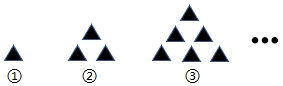$\text{A.}$ 10 $\text{B.}$ 15 $\text{C.}$ 18 $\text{D.}$ 21

B

#### 解析：

$\therefore$ 第(5)个图案中黑色三角形的个数为 $1+2+3+4+5=15$, 故选: $B$.
①点击 首页查看更多试卷和试题 , 点击查看 本题所在试卷